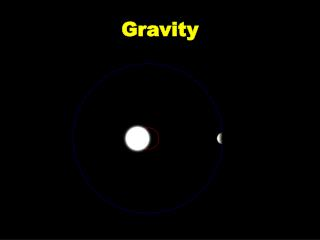Download PresentationGravity

Loading in 2 Seconds...

# Gravity - PowerPoint PPT Presentation

Gravity. Newton’s Universal Law of Gravitation. Newton’s conceptual leap in understanding gravity was his realization that the same force governs the motion of a falling object on Earth & the motion of the Moon in its orbit around the Earth. Universal Law of Gravitation.I am the owner, or an agent authorized to act on behalf of the owner, of the copyrighted work described.
Download Presentation## Gravity

An Image/Link below is provided (as is) to download presentation

Download Policy: Content on the Website is provided to you AS IS for your information and personal use and may not be sold / licensed / shared on other websites without getting consent from its author.While downloading, if for some reason you are not able to download a presentation, the publisher may have deleted the file from their server.

- - - - - - - - - - - - - - - - - - - - - - - - - - E N D - - - - - - - - - - - - - - - - - - - - - - - - - -
Presentation Transcript
1. Gravity

2. Newton’s Universal Law of Gravitation Newton’s conceptual leap in understandinggravity was his realizationthat the same force governs the motion of a falling objecton Earth & the motion of the Moon in its orbit around the Earth.

3. Universal Law of Gravitation • Every mass attracts every other mass through gravity. • The force of attraction between any 2 objects is directly proportional to the product of their masses. • Doubling the mass of 1object doubles Fg between the 2 objects.

4. Inverse Square • Doubling distance between two objects weakens force of gravity by a factor of 22 (or 4). • Fg drops off by ¼.

5. GMm Fg = r2 NEWTON’S UNIVERSALLAW OF GRAVITATION

6. G is universal gravitation constant.G = 6.67 x 10-11N m2. kg2.m1 m2 are mass of objects in kg.r is distance between ctrs m.

7. Gravity and Weight • Definition: The weight of an object is a measure of the Fg one object feels in the presence of another. • Weight Fg = mg. Where g is known.

8. Mass can be measured in 2 ways • Inertial mass - the mass of a body as determined by its momentum, as opposed to gravitational mass. How it accelerates • m = Fnet/a • Gravitational mass -the mass of a body determined by its response to the force of gravity.

9. 1. Prove that the acceleration of gravity g = GM/r2, where M is the mass of a planet or star r is its radius.

10. Fg = m2g = GM1m2. r2. g = GM1 is accl due to gravity r2. Mass of the object cancels out. Acceleration depends only on the mass of the planet (or star).

11. Questions: Raise Hands. • Is heavier body attracted to the Earth with more force than a light body. • Does the heavier object free fall faster? • Explain.

12. Newton’s Universal Law of Gravitation • Fg = GMm. R2. Describes Force btw point masses. What is a point mass?

13. Gravitational Field deals with space around the mass.

14. Gravitational Field Strength/Intensity -Force per unit mass experienced by a small “test mass” in space.

15. Gravitational Field = g.The value for acceleration, g, is also called the gravitational field. Set the weight equal the Fg. The units of the gravitational field are N/kg. Since mg = GMm. The small mass m cancels r2.Grav Field g = GM units N/kg. r2.Use to find acceleration or gravitational field.

16. 2. What is the gravitational field strength due to Earth at a point 450 km above the surface?

17. g = GM r2. • (6.67 x 10-11)(6 x 1024kg)(6.4 x 106m + 450 x 103m)2. • 4 x 1014.(6 850 000)2. • 4 x 1014. 4.7 x 1013. 8.5 N/kg

18. Fields are vectors. Forces resulting from more than 1 field is the sum of the field strengths.

19. Ex 3. Two spheres lie along a straight line. The sphere on the left has m = 1000-kg, and the right on the right has m = 100-kg. The distance between their centers is 5-m.Point A lies between the spheres at 2.5-m from the center of each.Calculate the field strength at point A.

20. 9.63 x 10 – 9 N/kg left

21. Rd Hamper 127 – 129 do pg 129 - 131.Purple boxes #1 – 5. Show all work.

22. Einstein vs. Newton9.5 min • http://www.youtube.com/watch?v=4yyb_RNJWUM

23. Orbits Maintained By Gravity

24. 4. Derive an equation to express the minimum velocity a satellite must have to stay in orbit.

25. Fg keeps the satellite in orbit. Set Fc equal to gravitational force. Fg = Fc GMem = mv2. r2 r v = (GMe)1/2. (r) 1/2.

26. 5. What is the velocity needed for a satellite to stay in orbit 200 km above the Earth’s surface?

27. First find the total distance between their centers.

28. 2.0 x 105 m. 6.37 x 106 m. 6.57 x 106m total r.

29. v = (GMe)1/2. (r) 1/2. (6.67x10-11)(5.98x1024 kg)1/2 (6.57 x 106m)1/2. 7790 m/s.

30. Cavendish Torsion Balancemeasurement of G.

31. Einstein vs. Newton9.5 min • http://www.youtube.com/watch?v=4yyb_RNJWUM

32. http://www.phy.ntnu.edu.tw/java/projectileOrbit/projectileOrbit.htmlhttp://www.phy.ntnu.edu.tw/java/projectileOrbit/projectileOrbit.html • Newton’s Cannon Launch Satellite • Apparent weightlessness. Satellites are in freefall around the Earth! That is why astronauts appear weightless. They are constantly falling toward Earth. Their rate of fall is balanced by their tangential momentum.

33. https://www.youtube.com/watch?v=EiljzeDL0co 45 minutes The universe season 2 Gravity, Roller Coasters, Space Travel & PEg.